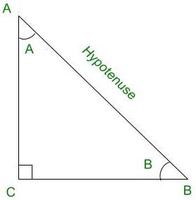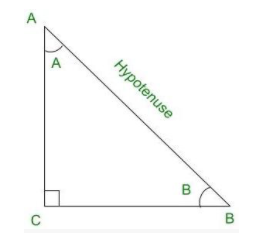Open in App
Not now

# If A and B are acute angles such that Sin A = Sin B, then prove that ∠A = ∠B

• Last Updated : 19 Nov, 2021

Trigonometry is the relation between the angles and sides of a right-angled triangle. In a right angles triangle, there are 3 angles of which one angle is a right angle (90°) and the other two angles are acute angles and there are 3 sides. The hypotenuse is the side opposite to the right angle and is the longest side in the right-angled triangle. There are two ways to determine the relationships among the three sides of the right-angled triangle, one is known as the Pythagoras theorem and the other method is through trigonometric ratios. Let’s take a look at what Trigonometric ratios are,

### The 6 Trigonometric ratios

The 6 trigonometric ratios are Sine (Sin), Cosine (cos), Tangent (Tan), Cosecant (Cosec), Secant (sec), Cotangent (cot). In the given right-angled triangle CBA below, the relation among AC, BC, and AB is provided with the help of these trigonometric ratios, lets learn the meaning and relations,Right-angled triangle CBA

Sine (sin)

Sine of an angle is defined by the ratio of lengths of sides which is opposite to the angle and the hypotenuse. For the above triangle, the value of angle sine is given for both ∠A and ∠B, the definition for sine angle is the ratio of the perpendicular to its hypotenuse.

SinA =SinB =Cosine (cos):

Cosine of an angle is defined by the ratio of lengths of sides which is adjacent to the angle and the hypotenuse. For the above triangle, the value of the angle cos is given for both ∠A and ∠B, the definition for cos angle is the ratio of the base to its hypotenuse.

CosA =CosB =Tangent (tan)

The tangent of an angle is defined by the ratio of length of sides which is opposite to the angle and the side which is adjacent to the angle. For the above triangle, the value of the angle tan is given for both ∠A and ∠B, the definition for tan angle is the ratio of the perpendicular to its base.

TanA =TanB =Cosecant (cosec)

The cosecant of an angle is defined by the ratio of the length of the hypotenuse and the side opposite the angle. For the above triangle, the value of the angle cosec is given for both ∠A and ∠B, the definition for cosec angle is the ratio of the hypotenuse to its perpendicular.

CosecA =CosecB =Secant (sec)

Secant of an angle is defined by the ratio of the length of the hypotenuse and the side and the side adjacent to the angle. For the above triangle, the value of the angle sec is given for both ∠A and ∠B, the definition for sec angle is the ratio of the hypotenuse to its base.

SecA =SecB =Cotangent (cot)

The cotangent of an angle is defined by the ratio of the length of sides that is adjacent to the angle and the side which is opposite to the angle. For the above triangle, the value of the angle cot is given for both ∠A and ∠B, the definition for cot angle is the ratio of the hypotenuse to its base.

CotA =CotB =### If ∠A and ∠B are acute angles such that sinA = sinB, then show that ∠A = ∠B.

It is a theorem as well, if the opposite sides of a triangle are equal then their opposite angles are also equal. In an isosceles triangle, the opposite sides and the opposite angles are equal. For the right-angled triangle, this can be easily proven through trigonometric ratios. From the diagram given below,sinA = Opposite side / Hypotenuse

= BC / AB   ⇢  (1)

sinB= Opposite side / Hypotenuse

= AC / AB   ⇢   (2)

It is given that,

sinA= sinB

Thus, from (1) and (2),

BC / AB = AC / AB

Therefore, BC = AC

In a triangle, if opposite sides are equal, then opposite angles are also equal.

Thus, ∠A = ∠B.

Hence proved.

### Similar Problems

Question 1: If ∠A and ∠B are acute angles such that cosA= cosB, then show that ∠A = ∠B.

Solution:

From the above diagram,

= AC / AB  ⇢  (1)

= BC/ AB   ⇢  (2)

It is given that,

cosA= cosB

Thus, from (1) and (2),

AC / AB = BC / AB

Therefore, AC = BC

In a triangle, if opposite sides are equal, then opposite angles are also equal.

Thus, ∠A = ∠B.

Hence proved.

Question 2: If ∠A and ∠B are acute angles such that cosecA= cosecB, then show that ∠A = ∠B.

Solution:

From the above diagram,

cosecA= Hypotenuse / Opposite side

= AB / BC  ⇢  (1)

cosecB= Hypotenuse / Opposite side

= AB / AC  ⇢  (2)

It is given that,

cosecA= cosecB

Thus, from (1) and (2),

AB / BC = AB / AC

Therefore, BC = AC

In a triangle, if opposite sides are equal, then opposite angles are also equal.

Hence proved.

Question 3: If ∠A and ∠B are acute angles such that secA= secB, then show that ∠A = ∠B.

Solution:

From the above diagram,

= AB / AC  ⇢  (1)

= AB / BC  ⇢  (2)

It is given that,

secA= secB

Thus, from (1) and (2),

AB / AC = AB / BC

Therefore, AC = BC

In a triangle, if opposite sides are equal, then opposite angles are also equal.

Hence proved.

My Personal Notes arrow_drop_up
Related Articles